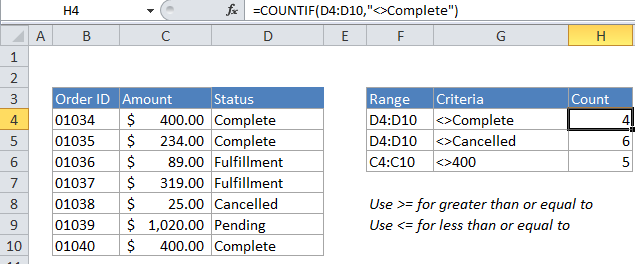## Excel Office

Excel How Tos, Tutorials, Tips & Tricks, Shortcuts

# Count cells not equal to in Excel

This tutorial shows how to Count cells not equal to in Excel using the example below;

## Formula

=COUNTIF(range,”<>X”)## Explanation

To count the number of cells that contain values not equal to a particular value, you can use the COUNTIF function. In the example above  X represents the value you don’t want to count. All other values will be counted.

Worked Example:   Highlight duplicate rows in Excel

In the example, the active cell contains this formula:

`=COUNTIF(D4:D10,"<>Complete")`

### How this formula works

COUNTIF counts the number of cells in the range that meet the criteria you supply.

In the example, we use “<>” (the logical operator for “does not equal”) to count cells in the range D4:D10 that don’t equal “complete”. COUNTIF returns the count as a result.

Worked Example:   Excel Data validation must begin with

COUNTIF is not case-sensitive. In this example, the word “complete” can appear in any combination of uppercase / lowercase letters and will not be counted.

Worked Example:   Count cells that contain text in Excel

If you want to use a value in another cell as part of the criteria, use the ampersand (&) character to concatenate like this:

`=COUNTIF(range,"<>"&a1)`

If the value in cell a1 is “100”, the criteria will be “<>100” after concatenation, and COUNTIF will count cells not equal to 100.